Function Repository Resource:

# InterpolatingFunctionDomain

Obtain the domain of an InterpolatingFunction object

Contributed by: Wolfram Staff
 ResourceFunction["InterpolatingFunctionDomain"][ifun] gives the domain for the InterpolatingFunction object ifun.

## Details and Options

ResourceFunction["InterpolatingFunctionDomain"] exposes the function by the same name from the package InterpolatingFunctionAnatomy.

## Examples

### Basic Examples (3)

Construct an InterpolatingFunction object and obtain its domain:

 In:=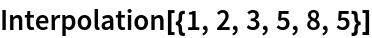Out=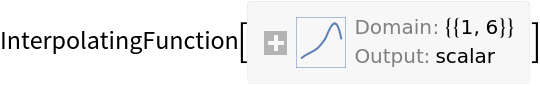In:=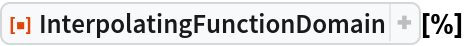Out=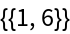Define a multidimensional interpolation function and find its domain:

 In:=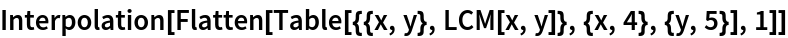Out=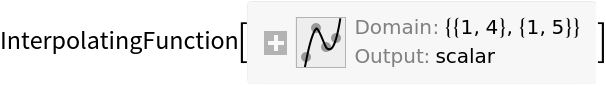In:=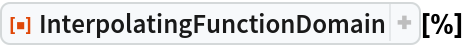Out=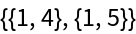Get a solution to a differential equation which cannot be computed up to the specified endpoint:

 In:=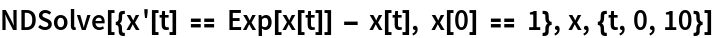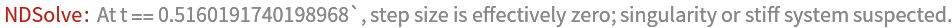Out=In:=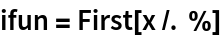Out=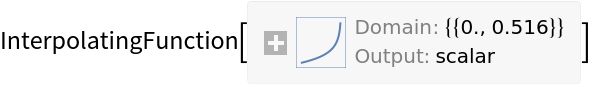Get its domain:

 In:=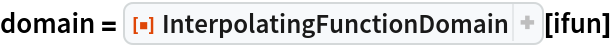Out=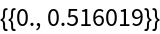Plot the solution over its domain:

 In:=Out=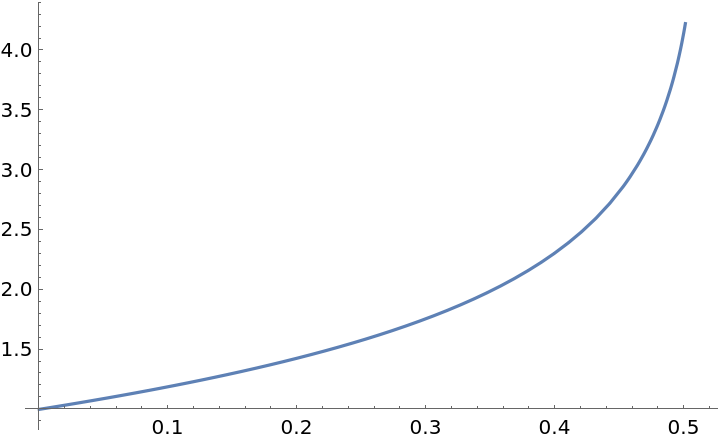### Properties and Relations (2)

The resource function InterpolatingFunctionData returns all the known properties of an InterpolatingFunction object, including its domain:

 In:=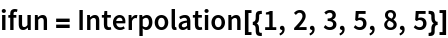Out=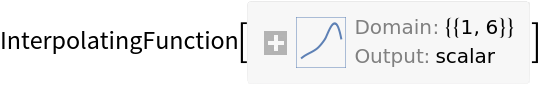In:=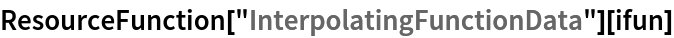Out=Compare with InterpolatingFunctionDomain:

 In:=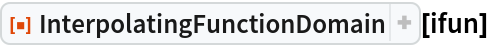Out=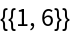## Version History

• 1.0.0 – 24 February 2020